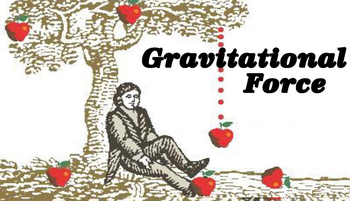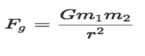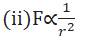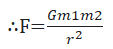# Gravitation﻿Introduction

-The force that holds us to  Earth, holds the Moon in orbit around Earth, holds Earth in orbit around the Sun and keeps the constituent of the universe bound together is known as gravitational force.

-It  is the force between two masses that exists universally among all bodies in the universe .

-Among four  fundamental  forces of nature ( strong force , electromagnetic  force  , weak force , and gravitational force ) it was the earliest one to be studied extensively.

-Until  17th century  the force that  causes  motion of planets and falling of objects was thought to be unrelated .In 1687 , Sir Isaac Newton published the basic  law  describing the force of gravitation which is called Newton’s Law of gravitation .  Using this law he was able to explain that the force which causes motion of planet and falling of object  is same and that’s gravitational force.

Newton’s Law of Gravitation

-It states that ”Every particle of the matter in the universe attracts every other particle with a force that is directly proportional to the product of masses of the particle and inversely proportional to the square of distance between them . ”Fig. The gravitational forces between two particles of masses m1 and m2 which are separated by distance ‘r’.

-According to this law , magnitude of force of gravitation (Fg) is determined by the following expression,Where,-It is known as universal law  because it holds true for every object in the universe without any exception.

Derivation

If F be the magnitude of  gravitational force between two particles of mass m1 and m2 separated by distance ‘r’ then , according to Newton’s law of gravitation,(i)F∝m1*m2Combining  equations (i) and (ii) , we get ,                fig. Gravitational force between two bodieswhere, G is the proportionality constant known as universal gravitational constant.

Gravitational constant (G)

-It is the gravitational force between two unit masses  separated by a unit distance .
- Its value was first determined experimentally by Henry Cavendish as- Dimensional  formula =-It is called universal constant because its value is same for any pair of masses and is independent of the nature of medium in which the masses are kept.
-It is also independent of
(a) chemical composition of the masses

(b) the temperature and pressure

( c) the direction of the gravitation forces between the masses

Characteristics of Gravitational force

-It is always attractive .
-It is independent of the medium.
-It is a conservative force i.e no  work done by gravity if a particle  moves in a closed path .
-It always acts along the line joining two masses.-It is a mutual force hence it is action-reaction force i.e force exerted by first particle on second particle is exactly equal and opposite to the force exerted by second particle on the first particle . Mathematically,-It is a central force  i.e it acts along the line joining the centers of the two interacting bodies .
-Gravitational force between two bodies does not depend upon the presence of other bodies between them.

Done with Newton's idea of Gravity, try that of Einstein: